# Torch-Struct: Structured Prediction Library¶A library of tested, GPU implementations of core structured prediction algorithms for deep learning applications.

• HMM / LinearChain-CRF

• HSMM / SemiMarkov-CRF

• Dependency Tree-CRF

• PCFG Binary Tree-CRF

Designed to be used as efficient batched layers in other PyTorch code.

Tutorial paper describing methodology.

## Getting Started¶

```!pip install -qU git+https://github.com/harvardnlp/pytorch-struct
# Optional CUDA kernels for FastLogSemiring
!pip install -qU git+https://github.com/harvardnlp/genbmm
# For plotting.
!pip install -q matplotlib
```
```import torch
from torch_struct import DependencyCRF, LinearChainCRF
import matplotlib.pyplot as plt
def show(x): plt.imshow(x.detach())
```
```# Make some data.
vals = torch.zeros(2, 10, 10) + 1e-5
vals[:, :5, :5] = torch.rand(5)
vals[:, 5:, 5:] = torch.rand(5)
dist = DependencyCRF(vals.log())
show(dist.log_potentials)
```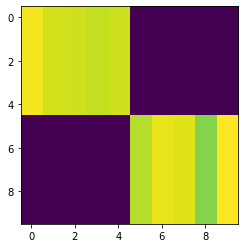```# Compute marginals
show(dist.marginals)
```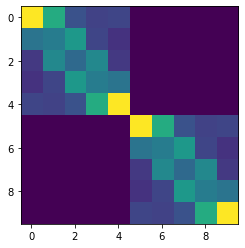```# Compute argmax
show(dist.argmax.detach())
```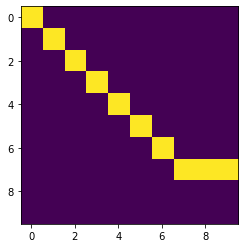```# Compute scoring and enumeration (forward / inside)
log_partition = dist.partition
max_score = dist.log_prob(dist.argmax)
```
```# Compute samples
show(dist.sample((1,)).detach()[0, 0])
```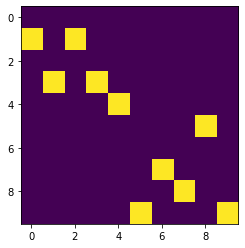```# Padding/Masking built into library.
dist = DependencyCRF(vals, lengths=torch.tensor([10, 7]))
show(dist.marginals)
plt.show()
show(dist.marginals)
```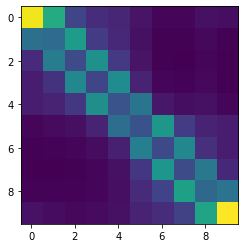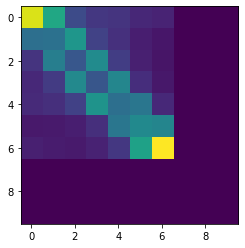```# Many other structured prediction approaches
chain = torch.zeros(2, 10, 10, 10) + 1e-5
chain[:, :, :, :] = vals.unsqueeze(-1).exp()
chain[:, :, :, :] += torch.eye(10, 10).view(1, 1, 10, 10)
chain[:, 0, :, 0] = 1
chain[:, -1,9, :] = 1
chain = chain.log()

dist = LinearChainCRF(chain)
show(dist.marginals.detach().sum(-1))
```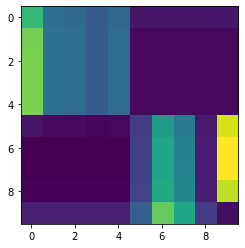## Library¶

Full docs: http://nlp.seas.harvard.edu/pytorch-struct/

Current distributions implemented:

• LinearChainCRF

• SemiMarkovCRF

• DependencyCRF

• NonProjectiveDependencyCRF

• TreeCRF

• NeuralPCFG / NeuralHMM

Each distribution includes:

• Argmax, sampling, entropy, partition, masking, log_probs, k-max

Extensions:

• Integration with `torchtext`, `pytorch-transformers`, `dgl`

• Adapters for generative structured models (CFG / HMM / HSMM)

• Common tree structured parameterizations TreeLSTM / SpanLSTM

## Low-level API:¶

Everything implemented through semiring dynamic programming.

• Log Marginals

• Max and MAP computation

• Sampling through specialized backprop

• Entropy and first-order semirings.

## Examples¶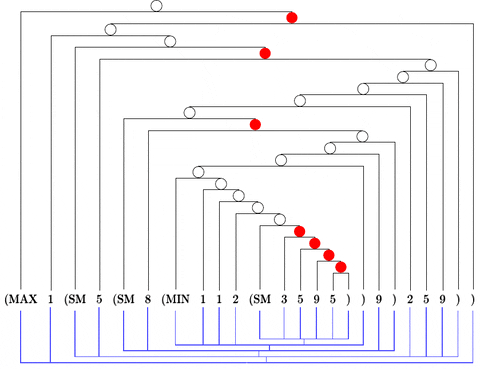## Citation¶

```@misc{alex2020torchstruct,
title={Torch-Struct: Deep Structured Prediction Library},
author={Alexander M. Rush},
year={2020},
eprint={2002.00876},
archivePrefix={arXiv},
primaryClass={cs.CL}
}
```

This work was partially supported by NSF grant IIS-1901030.